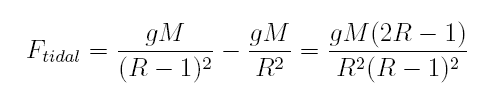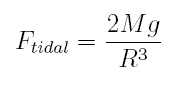Direct Observation of Moon Mass

Introduction

It is well known that the problem gets much worse when we include the sun's influence in the equations for the orbits of the three-body system. The equations of motion cannot be analytically solved, making the task of figuring out moon's mass of orbital measurements even more challenging . If we could observe a satellite under the influence of moon's gravity, the task is simplified tremendously . The mass of the satellite is negligible compared to the moon and the reduced mass of the system can just be approximated as satellite's mass. But, how would one figure out moon's mass before the era of satellites? Several clever methods do exist. One is based on determining the strength of the moon's tidal force.

Determination of Moon Mass using Tidal Forces

The expression for the moon's tidal force can be derived by considering moon's gravitational attraction . Let M be the moon's mass in the units of earth's mass. Let R be the distance to the moon in terms of the earth's radius. Let g be the acceleration on Earth's surface. Then, at the center of Earth, the acceleration due to the moon's attraction is gM/R2. At the sublunar surface, the force is gM/(R-1)2. The tidal force is the difference between the two accelerations:Since the distance between the earth and the moon is large compared to the radius of the earth, the equation above simplifies toIt's now clear how by measuring the tidal force on earth, it may be possible to determine the moon mass. But measuring this force presents a serious experimental challenge: we now know that it is roughly seven orders of magnitude less than g. The strength of the local weather conditions makes it impossible to use the terrestrial bodies of water for a precise measurement. It takes a brilliant experimenter to design an artificial system for such a delicate effect. In 1913, Albert Michelson found a way to do just that.

Michelson began by carefully leveling a cast-iron pipe with glass windows at each end. He then half-filled it with water and used a microscope to detect the changes in the water level. Despite the fact that the level changed by less than one thousandth of an inch, Michelson was able to make the measurement to the precision of one percent. In some versions of the experiment, Michelson varied the orientation of the pipes to measure the tidal forces in different directions. This aspect greatly resembles the Michelson-Morley experiment conducted six years earlier.

Despite the high precision of Michelson's experiment, the results are not sufficient to determine the mass of the moon with a high degree of accuracy. The derived mass of the moon differed by 30 percent from the contemporary accepted value. The discrepancy requires a careful explanation. The experiment assumes that earth's shape does not change due to the moon's tidal forces. This is, actually, incorrect. Its surface may rise and fall but as much as 9 inches as it is being deformed. We now know that the earth's rigidity roughly matches that of steel. This means that the earth is deformed by roughly 70 percent less than the same sized body made entirely of water. This explains the discrepancy in the calculated moon mass compared to the accepted value.

Conclusion

In this paper, we described Michelson's experiment of measuring the mass of the moon using the tidal forces. Michelson was able to measure the force with the precision of one percent. Despite of this, the mass of the moon could also be determined to within 30 percent of the contemporary accepted value. The discrepancy is explained by accounting for the earth's deformation under the influence of the tidal forces.

© 2007 Michael Ramm. The author grants permission to copy, distribute and display this work in unaltered form, with attribution to the author, for noncommercial purposes only. All other rights, including commercial rights, are reserved to the author.

References

 H. Goldstein, C. Poole, and J. Safko, Classical Mechanics (Addison-Wesley, 2002).

 A. Cook, The Motion of the Moon (Adam Hilger, 1988).

 Z. Kopal, An Introduction to the Study of the Moon (Reidel, 1966).

 R. B. Baldwin, A Fundamental Survey of the Moon (McGraw-Hill, 1965).